## – Find the missing X- and y-values and Pythagorean triples using the identity (x? y?)2 + (2xy)2 = (x² + y2)2. <

Question

Find the missing X- and y-values and Pythagorean triples using the identity

(x? y?)2 + (2xy)2 = (x² + y2)2.

Write the triples in parentheses with commas but no spaces between the values, and order the values

from least to greatest.

Type the correct answer in each box.

in progress 0
5 months 2021-08-09T07:53:55+00:00 1 Answers 1 views 0

1. Question:

Find the missing x- and y-values and Pythagorean triples using the identity given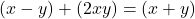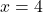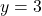Pythagorean Triples:

Write the triples in parentheses with commas but no spaces between the values, and order the values  from least to greatest.

Pythagorean triples is: (7, 24, 25)

Step-by-step explanation:

Pythagorean triples is expressed as: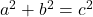In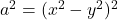Take square root of both sides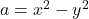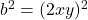Take square root of both sides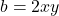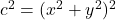Take square root of both sides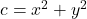So, we have:Pythagorean Triples: ?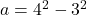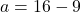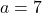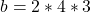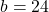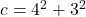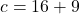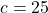Hence, the Pythagorean triples is: (7, 24, 25)